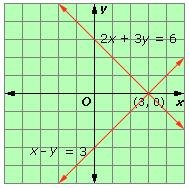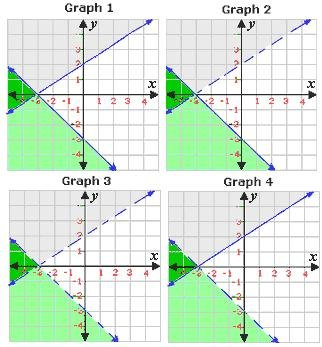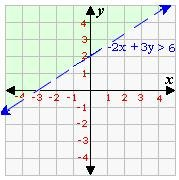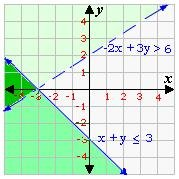Definition Of System Of Equations

A system of equations is a set of two or more equations with the same variables, graphed in the same coordinate plane

Examples of System of Equations

The figure below shows the graph of the system of equations
2x + 3y = 6 , x - y = 3

Video Examples: System of Equations centered cubic fccThier point of intersection gives the solution of the system of equations.

Solved Example on System of Equations

Ques: Which of the graphs represents the system of equations - 2x + 3y > 6 and x + y = - 3?A. Graph 1
B. Graph 2
C. Graph 3
D. Graph 4

Solution:

Step 1: Graph -2x + 3y > 6 on a coordinate plane.Step 2: Graph x + y ≤ -3 on the same coordinate plane.Step 3: The solutions are the coordinates of all the points in the region that is shaded in both colors.
Step 4: Check whether the solution (-4, 0) satisfies the two inequalities or not.
Step 5: -2x +3 3y > 6
-(-2) + 3 (0) > 6 [Replace x with -4 and y with 0.]
Step 6: 8 > 6 [ True]
Step 7: x + y ≤ -3
-4 + 0 ≤ - 3 [Replace x with -4 and y with 0.]
Step 8: -4 ≤ -3 [True.]
Step 9: Since the graph in step 2 matches with the graph 2 of choice B, Graph 2 represents the system of equations.